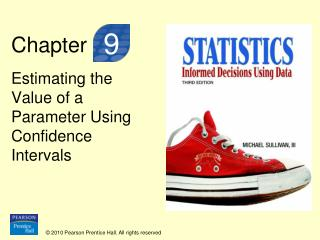DownloadDownload PresentationChapter

# Chapter

Télécharger la présentation## Chapter

- - - - - - - - - - - - - - - - - - - - - - - - - - - E N D - - - - - - - - - - - - - - - - - - - - - - - - - - -
##### Presentation Transcript

1. Chapter 9 Estimating the Value of a Parameter Using Confidence Intervals

2. Section 9.2 Confidence Intervals about a Population Mean When the Population Standard Deviation is Unknown

3. Objectives • Know the properties of Student’s t-distribution 2. Determine t-values 3. Construct and interpret a confidence interval for a population mean

4. Objective 1 • Know the Properties of Student’s t-Distribution

5. Student’s t-Distribution Suppose that a simple random sample of size n is taken from a population. If the population from which the sample is drawn follows a normal distribution, the distribution of follows Student’s t-distribution with n-1 degrees of freedom where is the sample mean and s is the sample standard deviation.

6. Parallel Example 1: Comparing the Standard Normal Distribution to the t-Distribution Using Simulation • Obtain 1,000 simple random samples of size n=5 from a normal population with =50 and =10. • Determine the sample mean and sample standard deviation for each of the samples. • Compute and for each sample. • Draw a histogram for both z and t.

7. Histogram for z

8. Histogram for t

9. CONCLUSIONS: • The histogram for z is symmetric and bell-shaped with the center of the distribution at 0 and virtually all the rectangles between -3 and 3. In other words, z follows a standard normal distribution. • The histogram for t is also symmetric and bell-shaped with the center of the distribution at 0, but the distribution of t has longer tails (i.e., t is more dispersed), so it is unlikely that t follows a standard normal distribution. The additional spread in the distribution of t can be attributed to the fact that we use s to find t instead of . Because the sample standard deviation is itself a random variable (rather than a constant such as ), we have more dispersion in the distribution of t.

10. Properties of the t-Distribution • The t-distribution is different for different degrees of freedom. • The t-distribution is centered at 0 and is symmetric about 0. • The area under the curve is 1. The area under the curve to the right of 0 equals the area under the curve to the left of 0 equals 1/2. • As t increases without bound, the graph approaches, but never equals, zero. As t decreases without bound, the graph approaches, but never equals, zero.

11. Properties of the t-Distribution • The area in the tails of the t-distribution is a little greater than the area in the tails of the standard normal distribution, because we are using s as an estimate of , thereby introducing further variability into the t- statistic. • As the sample size n increases, the density curve of t gets closer to the standard normal density curve. This result occurs because, as the sample size n increases, the values of s get closer to the values of , by the Law of Large Numbers.

12. Objective 2 • Determine t-Values

13. Parallel Example 2: Finding t-values Find the t-value such that the area under the t-distribution to the right of the t-value is 0.2 assuming 10 degrees of freedom. That is, find t0.20 with 10 degrees of freedom.

14. Solution The figure to the left shows the graph of the t-distribution with 10 degrees of freedom. The unknown value of t is labeled, and the area under the curve to the right of t is shaded. The value of t0.20with 10 degrees of freedom is 0.8791.

15. Objective 3 • Construct and Interpret a Confidence Interval for a Population Mean

16. Constructing a (1-)100% Confidence Interval for ,  Unknown Suppose that a simple random sample of size n is taken from a population with unknown mean  and unknown standard deviation . A (1-)100% confidence interval for  is given by Lower Upper bound: bound: Note: The interval is exact when the population is normally distributed. It is approximately correct for nonnormal populations, provided that n is large enough.

17. Parallel Example 3: Constructing a Confidence Interval about a Population Mean The pasteurization process reduces the amount of bacteria found in dairy products, such as milk. The following data represent the counts of bacteria in pasteurized milk (in CFU/mL) for a random sample of 12 pasteurized glasses of milk. Data courtesy of Dr. Michael Lee, Professor, Joliet Junior College. Construct a 95% confidence interval for the bacteria count.

18. NOTE: Each observation is in tens of thousand. So, 9.06 represents 9.06 x 104.

19. Solution: Checking Normality and Existence of Outliers Normal Probability Plot for CFU/ml

20. Solution: Checking Normality and Existence of Outliers Boxplot of CFU/mL

21. Lower bound: Upper bound: The 95% confidence interval for the mean bacteria count in pasteurized milk is (3.52, 9.30).

22. Parallel Example 5: The Effect of Outliers Suppose a student miscalculated the amount of bacteria and recorded a result of 2.3 x 105. We would include this value in the data set as 23.0. What effect does this additional observation have on the 95% confidence interval?

23. Solution: Checking Normality and Existence of Outliers Boxplot of CFU/mL

24. Solution Lower bound: Upper bound: The 95% confidence interval for the mean bacteria count in pasteurized milk, including the outlier is (3.86, 11.52).

25. CONCLUSIONS: • With the outlier, the sample mean is larger because the sample mean is not resistant • With the outlier, the sample standard deviation is larger because the sample standard deviation is not resistant • Without the outlier, the width of the interval decreased from 7.66 to 5.78.